Example Questions

Example Question #1 : Quadratic Equations

Solve: x2+6x+9=0

9

12

6

-3

3

-3

Explanation:

Given a quadratic equation equal to zero you can factor the equation and set each factor equal to zero. To factor you have to find two numbers that multiply to make 9 and add to make 6. The number is 3. So the factored form of the problem is (x+3)(x+3)=0. This statement is true only when x+3=0. Solving for x gives x=-3. Since this problem is multiple choice, you could also plug the given answers into the equation to see which one works.

Example Question #3 : How To Factor The Quadratic Equation

64x2 + 24x - 10 = 0

Solve for x

-1/4 and 3/4
-1/4 and -5/8
1/4 and -5/8
1/4 and -3/4
1/4 and 5/8
Explanation:

64x2 + 24x - 10 = 0

Factor the equation:

(8x + 5)(8x – 2) = 0

Set each side equal to zero

(8x + 5) = 0

x = -5/8

(8x – 2) = 0

x = 2/8 = 1/4

Example Question #31 : Quadratic Equation

All of the following functions have a exactly one root EXCEPT:

f(x) = 4x– 4x+1

f(x) = 9x2 – 6x + 4

f(x) = (1/4)x2 + x + 1

f(x) = (–1/9)x2 + 6x – 81

f(x) = x2 – 2x + 1

f(x) = 9x2 – 6x + 4

Explanation:

The roots of an equation are the points at which the function equals zero. We can set each function equal to zero and determine which functions have one root, and which does not.

Another piece of information will help. If a quadratic function has one root, then it must be a perfect square. This is because a quadratic function that is a perfect square can be written in the form (x – a)2. If we set (x – a)2 = 0 in order to find the root, we see that a is the only value that solves the equation, and thus a is the only root. Additionally, a quadratic equation is a perfect square if it can be written in the form a2x2 + 2abx + b2 = (ax + b)2

Let's examine the choice f(x) = 4x– 4x+1. To find the roots, we set f(x) = 0.

4x– 4x+1 = 0

We notice that 4x- 4x + 1 is a perfect square, since we could write it as (2x – 1)2. Thus, this equation has only one root, and it can't be the answer.

If we look at f(x) = x2 –2x + 1, we see that x2 – 2x + 1 is also a perfect square, because it could be written as (x – 1)2. This function also has a single root.

Next, we examine f(x) = (1/4)x2 + x + 1. Let us set f(x) = (1/4)x2 + x + 1 = 0.

(1/4)x2 + x + 1 = 0

We can multiply both sides by four to get rid of the fraction.

x+ 4x + 4 = 0

(x + 2)2 = 0

This function is also a perfect square and has a single root.

Now consider the choice f(x) = (–1/9)x2 + 6x – 81.

f(x) = (–1/9)x2 + 6x – 81 = 0

Multiply both sides by –9.

x– 54x + 729 = 0

(x – 27)2 = 0.

Finally, let's look at f(x) = 9x2 – 6x + 4. This CANNOT be written as a perfect square, because it is not in the form a2x2 + 2abx + b2 = (ax + b)2. It might be tempting to think that 9x2 - 6x + 4 = (3x - 2)2, but it does NOT, because (3x – 2)2 = 9x2 – 12x + 4. Therefore, because 9x2 – 6x + 4 is not a perfect square, it doesn't have exactly one root.

Example Question #4 : Quadratic Equations

The difference between a number and its square is 72. What is the number?

30

9

18

14

19

9

Explanation:

x2 – x = 72. Solve for x using the quadratic formula and x = 9 and –8. Only 9 satisfies the restrictions.

Example Question #1 : Quadratic Equations

Given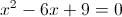and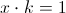, find the value of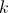.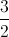Explanation:

We can factor the quadratic equation into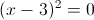.

Then we can see that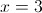.

Therefore,becomes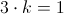and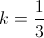.

Example Question #1 : Quadratic Equations

Which of the following is a root of the function?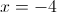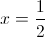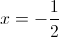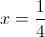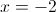Explanation:

The roots of a function are the x intercepts of the function. Whenever a function passes through a point on the x-axis, the value of the function is zero. In other words, to find the roots of a function, we must set the function equal to zero and solve for the possible values of x.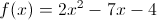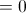This is a quadratic trinomial. Let's see if we can factor it. (We could use the quadratic formula, but it's easier to factor when we can.)

Because the coefficient in front of the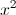is not equal to 1, we need to multiply this coefficient by the constant, which is –4. When we mutiply 2 and –4, we get –8. We must now think of two numbers that will multiply to give us –8, but will add to give us –7 (the coefficient in front of the x term). Those two numbers which multiply to give –8 and add to give –7 are –8 and 1. We will now rewrite –7x as –8x + x.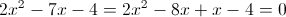We will then group the first two terms and the last two terms.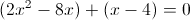We will next factor out a 2x from the first two terms.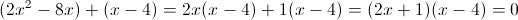Thus, when factored, the original equation becomes (2+ 1)(x – 4) = 0.

We now set each factor equal to zero and solve for x.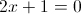Subtract 1 from both sides.

2x = –1

Divide both sides by 2.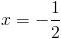Now, we set x – 4 equal to 0.

x – 4 = 0

x = 4

The roots of f(x) occur where x =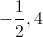.

The answer is therefore.

Example Question #1 : Quadratic Equations

36x2 -12x - 15 = 0

Solve for x

1/2 and 5/6

1/2 and 1/3

-1/2 and 5/6

-1/2 and -5/6

1/2 and -1/3

-1/2 and 5/6

Explanation:

36x2 - 12x - 15 = 0

Factor the equation:

(6x + 3)(6x - 5) = 0

Set each side equal to zero

6x + 3 = 0

x = -3/6 = -1/2

6x – 5 = 0

x = 5/6

Example Question #8 : Quadratic Equations

Find the roots of the equation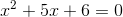.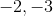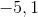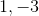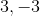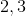Explanation:

To factor this, we need to find a pair of numbers that multiplies to 6 and sums to 5. The numbers 2 and 3 work. (2 * 3 = 6 and 2 + 3 = 5)

(x + 2)(x + 3) = 0

x = –2 or x = –3

Example Question #1 : Quadratic Equations

In the equation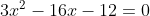what are the values of?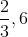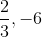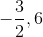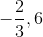Explanation:

To solve, begin by factoring the equation. We know that in our two factors, one will begin withand one will begin with. Fiddling with different factors of(we are looking for two numbers that, when multiplied byandseparately, will add to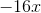), we come to the following: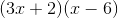(If you are unsure, double check by expanding the equation to match the original)

Now, set the each factor equal to 0: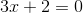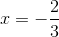Do the same for the second factor: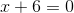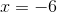Therefore, our two values ofare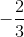and.

Alternatively, this problem can be solved by plugging each answer choice into the original equation and finding which set of numbers make the equation equate to 0.

Example Question #10 : Quadratic Equations

Solve for: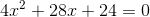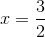and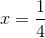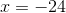and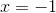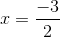and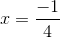and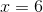andandExplanation:

To solve for, we need to factor the equation to identify its roots, or the values ofthat make the equation equal.We can factor out afrom each of the terms on the left side of the equation since they have that in common: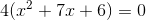Then, we can factor the remaining quadratic portion of the equation.andhave a difference of, suggesting that tryingandmight work: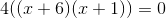At this point, we can identify the roots of the equation by settingand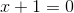and solving each of these equations to yieldand, respectively.